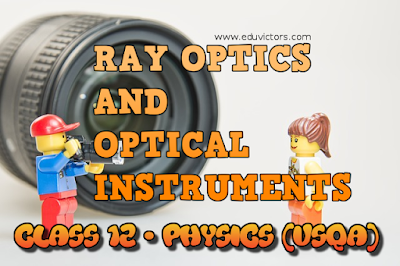## RAY OPTICS AND OPTICAL INSTRUMENTS

CBSE CLASS 12 - PHYSICS

Q1: What is the focal length and power of a plane glass plate?

Answer: Focal length of plane glass is infinite and its power = 0.

Q2: What is the mathematical relation between object distance (p) and image distance (i) in a plane mirror?

Q3: An object is placed at the focus of  concave lens. Where will its image be formed?

Answer: The real image will be formed at infinity.

Q4: If the speed of light were the same in all media, would refraction still occur when light passes from one medium to another?

Answer: No change in speed of light causes bending of light rays in refraction.

Q5(MCQ): A short pulse of white light is incident from air to a glass slab at normal incidence. After travelling through the slab, the first colour to emerge is

(a) blue
(b) green
(c) violet
(d) red

Q6: Name the branch of physics for measurement of light energy.

Q7: When does a concave mirror for a virtual image?

Answer: When object is placed between its pole and focus.

Q8: What is focal plane?

Answer: In case when parallel ray are not parallel to principal axis, they intersect at a point which is not on the axis. Plane through this point is called focal plane.

Q9: What type of images are formed by convex mirror?

Answer: A convex mirror can form only a virtual image.

Q10: Out of concave or convex mirror of same focal length, which has smaller field of view?

Q11: Write any two applications of concave mirror?

Used by dental surgeons to view dental cavities
As shaving mirror.

Q12: Mention any two applications of convex mirror.

Used as rear view mirror in cars
Used in anti shoplifting devices.

Q13: Define internal reflection.

Answer: When light travels from an optically denser medium to rarer medium at the interface, it is partially reflected back into the same medium and partly refracted to the same medium. This reflection is called internal reflection.

Q14: Define the laws of reflection.

Angle of incidence = angle of reflection i.e. (∠i = ∠ r)
Incident ray, normal ray and reflected ray lie in the same plane.

Q15: If a ray is incident on a plane mirror at an angle of incidence i,  how much will be the deviation?

Q16: What will be the minimum size of a plane mirror fixed on a wall of room, so that a person at the centre pf room may see the full image of the wall behind him?

Answer: It should be ⅓rd the size of the wall.

Q17: The shortest height of a vertical mirror required to see the entire image of a man will be _____?